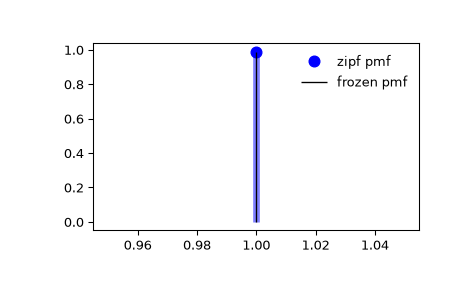# scipy.stats.zipf¶

scipy.stats.zipf = <scipy.stats._discrete_distns.zipf_gen object>[source]

A Zipf (Zeta) discrete random variable.

As an instance of the rv_discrete class, zipf object inherits from it a collection of generic methods (see below for the full list), and completes them with details specific for this particular distribution.

Notes

The probability mass function for zipf is:

$f(k, a) = \frac{1}{\zeta(a) k^a}$

for $$k \ge 1$$, $$a > 1$$.

zipf takes $$a > 1$$ as shape parameter. $$\zeta$$ is the Riemann zeta function (scipy.special.zeta)

The Zipf distribution is also known as the zeta distribution, which is a special case of the Zipfian distribution (zipfian).

The probability mass function above is defined in the “standardized” form. To shift distribution use the loc parameter. Specifically, zipf.pmf(k, a, loc) is identically equivalent to zipf.pmf(k - loc, a).

References

1

“Zeta Distribution”, Wikipedia, https://en.wikipedia.org/wiki/Zeta_distribution

Examples

>>> from scipy.stats import zipf
>>> import matplotlib.pyplot as plt
>>> fig, ax = plt.subplots(1, 1)


Calculate the first four moments:

>>> a = 6.5
>>> mean, var, skew, kurt = zipf.stats(a, moments='mvsk')


Display the probability mass function (pmf):

>>> x = np.arange(zipf.ppf(0.01, a),
...               zipf.ppf(0.99, a))
>>> ax.plot(x, zipf.pmf(x, a), 'bo', ms=8, label='zipf pmf')
>>> ax.vlines(x, 0, zipf.pmf(x, a), colors='b', lw=5, alpha=0.5)


Alternatively, the distribution object can be called (as a function) to fix the shape and location. This returns a “frozen” RV object holding the given parameters fixed.

Freeze the distribution and display the frozen pmf:

>>> rv = zipf(a)
>>> ax.vlines(x, 0, rv.pmf(x), colors='k', linestyles='-', lw=1,
...         label='frozen pmf')
>>> ax.legend(loc='best', frameon=False)
>>> plt.show()Check accuracy of cdf and ppf:

>>> prob = zipf.cdf(x, a)
>>> np.allclose(x, zipf.ppf(prob, a))
True


Generate random numbers:

>>> r = zipf.rvs(a, size=1000)


Confirm that zipf is the large n limit of zipfian.

>>> from scipy.stats import zipfian
>>> k = np.arange(11)
>>> np.allclose(zipf.pmf(k, a), zipfian.pmf(k, a, n=10000000))
True


Methods

 rvs(a, loc=0, size=1, random_state=None) Random variates. pmf(k, a, loc=0) Probability mass function. logpmf(k, a, loc=0) Log of the probability mass function. cdf(k, a, loc=0) Cumulative distribution function. logcdf(k, a, loc=0) Log of the cumulative distribution function. sf(k, a, loc=0) Survival function (also defined as 1 - cdf, but sf is sometimes more accurate). logsf(k, a, loc=0) Log of the survival function. ppf(q, a, loc=0) Percent point function (inverse of cdf — percentiles). isf(q, a, loc=0) Inverse survival function (inverse of sf). stats(a, loc=0, moments=’mv’) Mean(‘m’), variance(‘v’), skew(‘s’), and/or kurtosis(‘k’). entropy(a, loc=0) (Differential) entropy of the RV. expect(func, args=(a,), loc=0, lb=None, ub=None, conditional=False) Expected value of a function (of one argument) with respect to the distribution. median(a, loc=0) Median of the distribution. mean(a, loc=0) Mean of the distribution. var(a, loc=0) Variance of the distribution. std(a, loc=0) Standard deviation of the distribution. interval(alpha, a, loc=0) Endpoints of the range that contains fraction alpha [0, 1] of the distribution